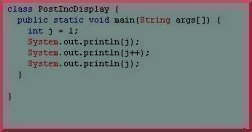•• # Postfix

The sum of X and Y is written as X+Y where + is the operator while X and Y are the operands. We have always learnt to write the sum of two numbers as X + Y; this notation is know as infix. Here, we’ll be talking about the postfix notation of representing arithmetic operations.

XY+ // postfix

The relative position of the operator with respect to the operands tells whether the expression is written in postfix or infix notation. As the above expression shows, when the position of the operator is after the two operands then the expression is said to be in postfix notation and if the position of the operator is between the two operands the expression is in infix notation.## Rules for Converting Infix to Postfix

Here are some rules for converting infix to postfix:

1. If the expression contains any parentheses, then they should be converted first.
2. Conversion should be done according to the DMAS rule with precedence given first to division (/), then multiplication (*), then addition (+) and finally subtraction (-). That is, in an expression containing /, *, + and – first the division (/) sign should be evaluated followed by multiplication (*), addition (+) and subtraction (-). Also, the exponentiation operator has a precedence higher than these four common operators.
3. If there are two operators of the same precedence, then conversion should be done left to right.

These rules will become clearer with some examples.

## Examples

Let us now consider some additional examples. The expression given below has a combination of parentheses and division, multiplication and addition operators. In the first step according to rule number 1 the expression inside the parentheses is evaluated and converted into postfix notation, after this according to rule number 2 first division is evaluated followed by multiplication and then addition.

 A + (B * C) * D / E expression containing parentheses A + (BC *) * D / E convert the multiplication A + (BC *) * D E / convert the division A + (BC*) D E / * convert the multiplication A (BC*) D E / * + convert the addition ABC*D*E/+ postfix form

The main thing that should be remembered during the conversion process is that the operator with highest precedence is converted first and that the portion of expression already converted is treated as one single operand.

Here’s another example.

Note: In this example, the parentheses have deliberately been added to reverse the precedence.

 (A + B) * C infix form (AB +) * C convert the addition (AB +) C * convert the multiplication AB + C* postfix form

In the above example, addition is converted before the multiplication, this is because the parentheses around A+B gives + more precedence than *. After the conversion of A+ B, AB+ is treated as one single operand and not a combination of operands and operator. The rules for converting infix to postfix are simple, providing that the order of precedence is known.

According to rule number 3, when unparenthesized operators of the same precedence are scanned, the order of conversion is left to right except in the case of exponentiation, where the order is assumed to be from right to left. Thus A + B + C means (A + B) + C, whereas A \$ B \$ C means A \$ (B \$ C). By using parentheses we can override the default precedence.

The following are some ore examples of infix expressions and their postfix equivalents.

Infix Postfix
A + B AB+
A + B – C + D AB+CD+-
(A + B) * (C – D) AB+ CD -*
A\$B*C-D+E/F/(G + H) AB\$C*D-EF/GH+/+
A – B/(C*D\$E) ABCDE\$*/-
1. #### gaurav kauhsik

8 June, 2012 at 5:15 pm

postfix of A part is: ABC+*D-E*F/
part B: AB-C*D/FG+*
its just a try from my side may be correct or not..:)

10 March, 2012 at 3:48 am

Please convert to post fix and prefix:

a. (A*(B+C)-D*E)/F

B. (A-B)*C/D*F+G

3. #### Felix

20 January, 2012 at 10:52 am

A (BC*) D E / * +convert the additionABC*D*E/+postfix formHow did the last step occur(i.e. how did the * symbol come in between D and E)? It would be helpful if someone could explain.

4. #### virendra

23 February, 2011 at 3:16 pm

(a+b)*c/d plesae give answer to postfix exprision?????????

• #### Taranbir singh

10 February, 2012 at 8:15 am

(a+b)*c/d postfix will be:
(ab+)*cd/
ab+cd/*

5. #### Daniel Memenode

30 November, 2010 at 6:46 pm

Originally posted by Vatra: “You have an error in the fourth example:
infix: A\$B*C-D+E/F/(G + H)
wrong postfix: AB\$C*DEF/GH+/+-
correct postfix: AB\$C*D-EF/GH+/+

The error is that when you have two signs of the same precedence, you place the one on the stack in the postfix.”

Thanks, fixed. If anyone believes this is incorrect let us know. I’m not the author of the above article.